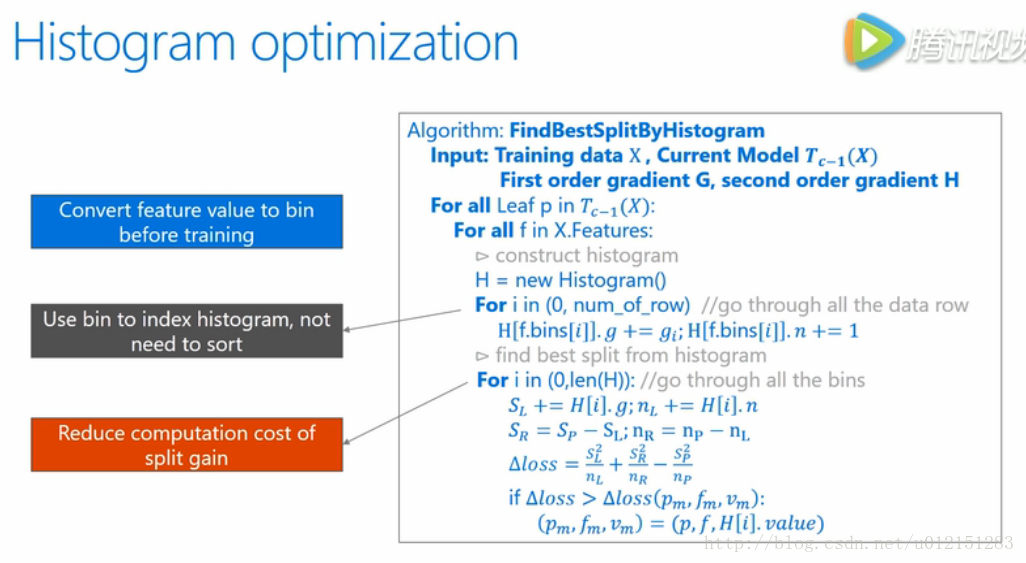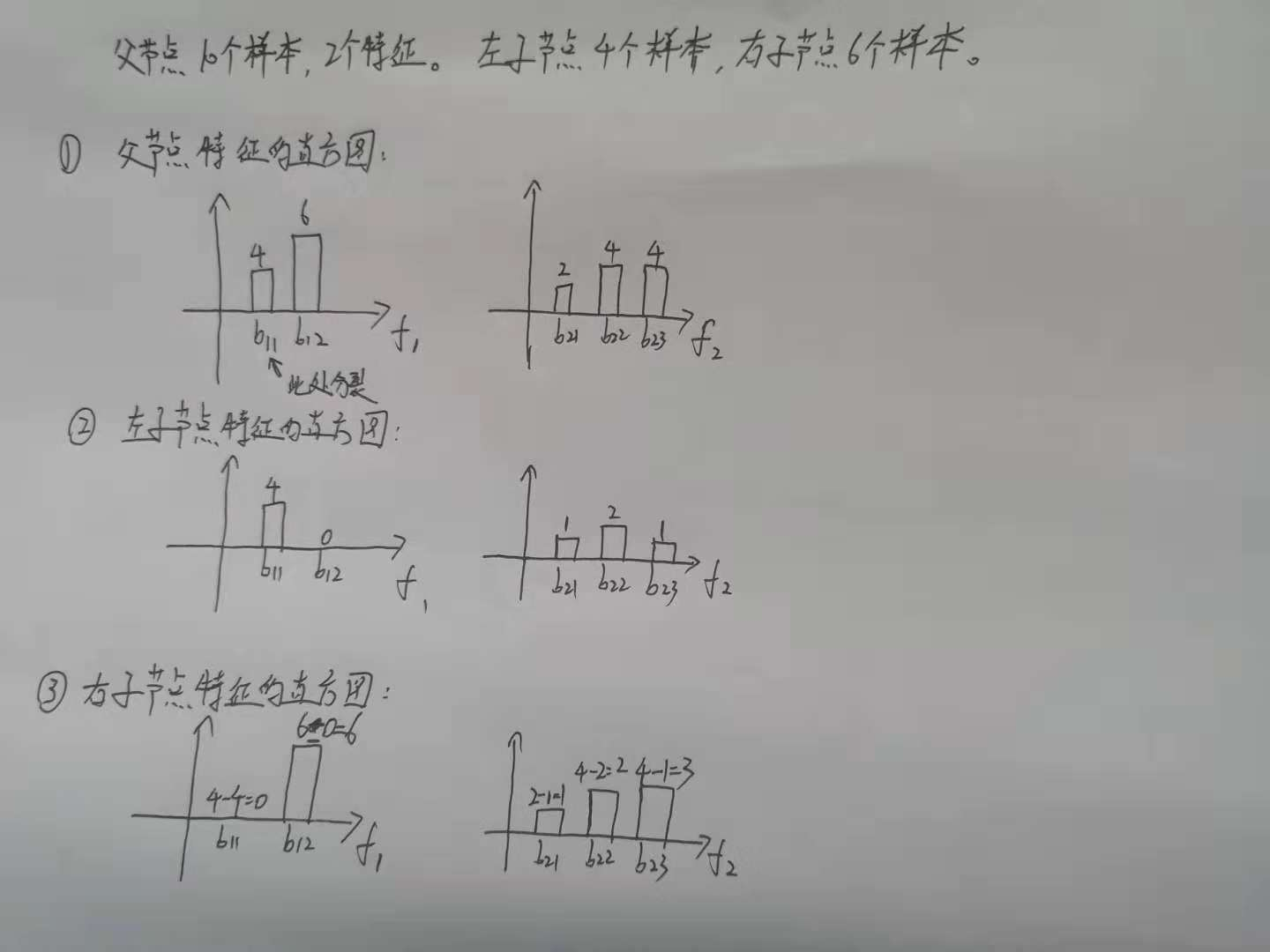# 一、概述

histogram算法简单来说，就是先对特征值进行装箱处理，形成一个一个的bins。对于连续特征来说，装箱处理就是特征工程中的离散化：如[0,0.3)—>0，[0.3,0.7)—->1等。在Lightgbm中默认的#bins为256（1个字节的能表示的长度，可以设置）。对于分类特征来说，则是每一种取值放入一个bin，且当取值的个数大于max bin数时，会忽略那些很少出现的category值。

# 二、算法流程# 三、源码分析

①如何将特征映射到bin呢？即如何分桶？对于连续特征和类别特征分别怎么样处理？

②如何构建直方图？直方图算法累加的g是什么？难道没有二阶导数h吗？

## 3.1 特征分桶

### 3.1.1 连续特征

bin.cpp中，我们可以看到GreedyFindBin函数和FindBinWithZeroAsOneBin函数，这两个函数得到了数值型特征取值（负数，0，正数）的各个bin的切分点，即bin_upper_bound。

/************************************************************************************
****************************得到数值型特征取值的各个bin的切分点*************************
*************************************************************************************/
std::vector<double> GreedyFindBin(const double* distinct_values, const int* counts,
int num_distinct_values, int max_bin, size_t total_cnt, int min_data_in_bin) {
// counts为特征取值计数的数组；
//distinct_values为特征的不同的取值的数组；num_distinct_values为特征有多少个不同的取值。

// bin_upper_bound就是记录桶分界的数组
std::vector<double> bin_upper_bound;
CHECK(max_bin > 0);

// 特征取值数比max_bin数量少，直接取distinct_values的中点放置
if (num_distinct_values <= max_bin) {
bin_upper_bound.clear();
int cur_cnt_inbin = 0;
for (int i = 0; i < num_distinct_values - 1; ++i) {
cur_cnt_inbin += counts[i];
// min_data_in_bin默认为3。
// 若一个特征的取值比min_data_in_bin小，则累积下一个取值，直到比min_data_in_bin大，进入循环。
if (cur_cnt_inbin >= min_data_in_bin) {
// 取当前值和下一个值的均值作为该桶的分界点bin_upper_bound
auto val = Common::GetDoubleUpperBound((distinct_values[i] + distinct_values[i + 1]) / 2.0);
// CheckDoubleEqualOrdered返回真的条件：val<bin_upper_bound.back()。注意前面有个！。
if (bin_upper_bound.empty() || !Common::CheckDoubleEqualOrdered(bin_upper_bound.back(), val)) {
bin_upper_bound.push_back(val); //插入到最后
cur_cnt_inbin = 0;
}
}
}
// 对于最后一个桶的上界则为无穷大
cur_cnt_inbin += counts[num_distinct_values - 1];
bin_upper_bound.push_back(std::numeric_limits<double>::infinity());
}
// 特征取值数比max_bin来得大，说明几个特征值要共用一个bin
else {
if (min_data_in_bin > 0) {
max_bin = std::min(max_bin, static_cast<int>(total_cnt / min_data_in_bin));
max_bin = std::max(max_bin, 1);
}
// mean size for one bin
double mean_bin_size = static_cast<double>(total_cnt) / max_bin;

int rest_bin_cnt = max_bin;
int rest_sample_cnt = static_cast<int>(total_cnt);
// 定义is_big_count_value数组：初始设定特征每一个不同的值的数量都小（false）。
std::vector<bool> is_big_count_value(num_distinct_values, false);

// 如果一个特征值的数目比mean_bin_size大，那么这些特征需要单独一个bin
for (int i = 0; i < num_distinct_values; ++i) {
// 如果一个特征值的数目比mean_bin_size大，则设定这个特征值对应的is_big_count_value为真。。
if (counts[i] >= mean_bin_size) {
is_big_count_value[i] = true;
--rest_bin_cnt;
rest_sample_cnt -= counts[i];
}
}

// 剩下的特征取值的样本数平均每个剩下的bin：mean size for one bin
mean_bin_size = static_cast<double>(rest_sample_cnt) / rest_bin_cnt;
std::vector<double> upper_bounds(max_bin, std::numeric_limits<double>::infinity());
std::vector<double> lower_bounds(max_bin, std::numeric_limits<double>::infinity());

int bin_cnt = 0;
lower_bounds[bin_cnt] = distinct_values;
int cur_cnt_inbin = 0;
// 重新遍历所有的特征值（包括数目大和数目小的）
for (int i = 0; i < num_distinct_values - 1; ++i) {
// 如果当前的特征值数目是小的
if (!is_big_count_value[i]) {
rest_sample_cnt -= counts[i];
}
cur_cnt_inbin += counts[i];

// 若cur_cnt_inbin太少，则累积下一个取值，直到满足条件，进入循环。
// need a new bin 当前的特征如果是需要单独成一个bin，或者当前几个特征计数超过了mean_bin_size，或者下一个是需要独立成桶的
if (is_big_count_value[i] || cur_cnt_inbin >= mean_bin_size ||
(is_big_count_value[i + 1] && cur_cnt_inbin >= std::max(1.0, mean_bin_size * 0.5f))) {
upper_bounds[bin_cnt] = distinct_values[i]; // 第i个bin的最大就是 distinct_values[i]了
++bin_cnt;
lower_bounds[bin_cnt] = distinct_values[i + 1]; //下一个bin的最小就是distinct_values[i + 1]，注意先++bin了
if (bin_cnt >= max_bin - 1) { break; }
cur_cnt_inbin = 0;
if (!is_big_count_value[i]) {
--rest_bin_cnt;
mean_bin_size = rest_sample_cnt / static_cast<double>(rest_bin_cnt);
}
}
}
++bin_cnt;
// update bin upper bound 与特征取值数比max_bin数量少的操作类似，取当前值和下一个值的均值作为该桶的分界点
bin_upper_bound.clear();
for (int i = 0; i < bin_cnt - 1; ++i) {
auto val = Common::GetDoubleUpperBound((upper_bounds[i] + lower_bounds[i + 1]) / 2.0);
if (bin_upper_bound.empty() || !Common::CheckDoubleEqualOrdered(bin_upper_bound.back(), val)) {
bin_upper_bound.push_back(val);
}
}
// last bin upper bound
bin_upper_bound.push_back(std::numeric_limits<double>::infinity());
}
// bin_upper_bound即数值型特征取值的各个bin的切分点
return bin_upper_bound;
}

/************************************************************************************
********************得数值型特征取值（负数，0，正数）的各个bin的切分点*******************
*************************************************************************************/
std::vector<double> FindBinWithZeroAsOneBin(const double* distinct_values, const int* counts,
int num_distinct_values, int max_bin, size_t total_sample_cnt, int min_data_in_bin) {
std::vector<double> bin_upper_bound;
// left_cnt_data记录小于0的值
int left_cnt_data = 0;
int cnt_zero = 0;
// right_cnt_data记录大于0的值
int right_cnt_data = 0;
for (int i = 0; i < num_distinct_values; ++i) {
//  double kZeroThreshold = 1e-35f
if (distinct_values[i] <= -kZeroThreshold) {
left_cnt_data += counts[i];
} else if (distinct_values[i] > kZeroThreshold) {
right_cnt_data += counts[i];
} else {
cnt_zero += counts[i];
}
}

//如果特征值里存在0和正数，则left_cnt不为-1，则left_cnt是最后一个负数的位置
int left_cnt = -1;
for (int i = 0; i < num_distinct_values; ++i) {
if (distinct_values[i] > -kZeroThreshold) {
left_cnt = i;
break;
}
}

// 如果特征值全是负值，left_cnt = num_distinct_values
if (left_cnt < 0) {
left_cnt = num_distinct_values;
}

if (left_cnt > 0) {
// 负数除以（正数+负数）的比例，即负数的桶数。-1的1就是0的桶。
int left_max_bin = static_cast<int>(static_cast<double>(left_cnt_data) / (total_sample_cnt - cnt_zero) * (max_bin - 1));
left_max_bin = std::max(1, left_max_bin);
bin_upper_bound = GreedyFindBin(distinct_values, counts, left_cnt, left_max_bin, left_cnt_data, min_data_in_bin);
// 负数桶的分界点最后一个自然是-kZeroThreshold
bin_upper_bound.back() = -kZeroThreshold;
}

//如果特征值存在正数，则right_start不为-1，则right_start是第一个正数开始的位置
int right_start = -1;
for (int i = left_cnt; i < num_distinct_values; ++i) {
if (distinct_values[i] > kZeroThreshold) {
right_start = i;
break;
}
}
// 如果特征值里存在正数
if (right_start >= 0) {
int right_max_bin = max_bin - 1 - static_cast<int>(bin_upper_bound.size());
CHECK(right_max_bin > 0);
auto right_bounds = GreedyFindBin(distinct_values + right_start, counts + right_start,
num_distinct_values - right_start, right_max_bin, right_cnt_data, min_data_in_bin);
// 正数桶的分界点第一个自然是kZeroThreshold，拼接到了-kZeroThreshold后面。
bin_upper_bound.push_back(kZeroThreshold);
// 插入正数桶的分界点，形成最终的分界点数组。
bin_upper_bound.insert(bin_upper_bound.end(), right_bounds.begin(), right_bounds.end());
} else {
bin_upper_bound.push_back(std::numeric_limits<double>::infinity());
}

// bin_upper_bound即数值型特征取值（负数，0，正数）的各个bin的切分点
return bin_upper_bound;
}


### 3.1.2 类别特征

    //从小到大排序values数组，从第0个到num_sample_values个。
std::stable_sort(values, values + num_sample_values);

// push zero in the front
// 如果最小的特征值大于0且存在0，则把0放到distinct_values的第一位。或者全部是NaN数据。
if (num_sample_values == 0 || (values > 0.0f && zero_cnt > 0)) {
distinct_values.push_back(0.0f);
counts.push_back(zero_cnt);
}

if (num_sample_values > 0) {
distinct_values.push_back(values);
counts.push_back(1);
}

for (int i = 1; i < num_sample_values; ++i) {
// 如果values[i - 1]小于values[i]
if (!Common::CheckDoubleEqualOrdered(values[i - 1], values[i])) {
if (values[i - 1] < 0.0f && values[i] > 0.0f) {
distinct_values.push_back(0.0f);
counts.push_back(zero_cnt);
}
distinct_values.push_back(values[i]);
counts.push_back(1);
} else {
// use the large value
// 如果values[i - 1]不小于values[i]，即只可能values[i - 1]等于values[i]，说明distinct_values已经有了这个值了，只需要把它的counts加1.
distinct_values.back() = values[i];
++counts.back();
}
}


// sort by counts 特征取值按出现的次数排序（大到小）
Common::SortForPair<int, int>(counts_int, distinct_values_int, 0, true);
// avoid first bin is zero
if (distinct_values_int == 0) {
if (counts_int.size() == 1) {
counts_int.push_back(0);
distinct_values_int.push_back(distinct_values_int + 1);
}
// 交换counts_int和counts_int的值
std::swap(counts_int, counts_int);
std::swap(distinct_values_int, distinct_values_int);
}
// will ignore the categorical of small counts
int cut_cnt = static_cast<int>((total_sample_cnt - na_cnt) * 0.99f);
size_t cur_cat = 0;
// categorical_2_bin_ (unordered_map类型) 将特征取值到哪个bin和一一对应起来
categorical_2_bin_.clear();
// bin_2_categorical_（vector类型）记录bin对应的特征取值
bin_2_categorical_.clear();
int used_cnt = 0;
max_bin = std::min(static_cast<int>(distinct_values_int.size()), max_bin);
cnt_in_bin.clear();
// 类别特征值已经按数量从大到小排列，累积特征值的数目，放弃后1%的类别特征值，即忽略一些出现次数很少的特征取值
while (cur_cat < distinct_values_int.size()
&& (used_cnt < cut_cnt || num_bin_ < max_bin)) {
if (counts_int[cur_cat] < min_data_in_bin && cur_cat > 1) {
break;
}
//为bin_2_categorical_和categorical_2_bin_赋值
bin_2_categorical_.push_back(distinct_values_int[cur_cat]);
categorical_2_bin_[distinct_values_int[cur_cat]] = static_cast<unsigned int>(num_bin_);
used_cnt += counts_int[cur_cat];
cnt_in_bin.push_back(counts_int[cur_cat]);
++num_bin_;
++cur_cat;
}


### 3.1.3 总结

FindBin→FindBinWithZeroAsOneBin→GreedyFindBin

## 3.2 构建直方图

ConstructHistogram函数即为关键代码如下（这个函数出现在很多文件中）：

  void ConstructHistogram(const data_size_t* data_indices, data_size_t num_data,
const score_t* ordered_gradients, const score_t* ordered_hessians,
HistogramBinEntry* out) const override {
// num_data与0011进行与，即num_data不会大于3
const data_size_t rest = num_data & 0x3;
data_size_t i = 0;
for (; i < num_data - rest; i += 4) {
const VAL_T bin0 = data_[data_indices[i]];
const VAL_T bin1 = data_[data_indices[i + 1]];
const VAL_T bin2 = data_[data_indices[i + 2]];
const VAL_T bin3 = data_[data_indices[i + 3]];

out[bin0].sum_hessians += ordered_hessians[i];
out[bin1].sum_hessians += ordered_hessians[i + 1];
out[bin2].sum_hessians += ordered_hessians[i + 2];
out[bin3].sum_hessians += ordered_hessians[i + 3];

++out[bin0].cnt;
++out[bin1].cnt;
++out[bin2].cnt;
++out[bin3].cnt;
}
for (; i < num_data; ++i) {
const VAL_T bin = data_[data_indices[i]];
out[bin].sum_hessians += ordered_hessians[i];
++out[bin].cnt;
}
}


# 四、histogram算法与 pre-sorted算法对比

## 3.1 优势

• Pre-sorted 算法需要的内存约是训练数据的两倍(2 * #data * #features* 4Bytes)，它需要用32位浮点(4Bytes)来保存 feature value，并且对每一列特征，都需要一个额外的排好序的索引，这也需要32位(4Bytes)的存储空间。因此是(2 * #data * #features* 4Bytes)。而对于 histogram 算法，则只需要(#data * #features * 1Bytes)的内存消耗，仅为 pre-sorted算法的1/8。因为 histogram 算法仅需要存储 feature bin value (离散化后的数值)，不需要原始的 feature value，也不用排序，而 bin value 用 1Bytes(256 bins) 的大小一般也就足够了。

• 计算上的优势则是大幅减少了计算分割点增益的次数。对于每一个特征，pre-sorted 需要对每一个不同特征值都计算一次分割增益，代价是O(#feature*#distinct_values_of_the_feature)；而 histogram 只需要计算#bins次，代价是(#feature*#bins)。

• 还有一个很重要的点是cache-miss。事实上，cache-miss对速度的影响是特别大的。预排序中有2个操作频繁的地方会造成cache miss，一是对梯度的访问，在计算gain的时候需要利用梯度，不同特征访问梯度的顺序都是不一样的，且是随机的，因此这部分会造成严重的cache-miss。二是对于索引表的访问，预排序使用了一个行号到叶子节点号的索引表（row_idx_to_tree_node_idx ），来防止数据切分时对所有的数据进行切分，即只对该叶子节点上的样本切分。在与level-wise进行结合的时候， 每一个叶子节点都要切分数据，这也是随机的访问。这样会带来严重的系统性能下降。而直方图算法则是天然的cache friendly。在直方图算法的第3个for循环的时候，就已经统计好了每个bin的梯度，因此，在计算gain的时候，只需要对bin进行访问，造成的cache-miss问题会小很多。

• 最后，在数据并行的时候，用 histgoram 可以大幅降低通信代价。用 pre-sorted 算法的话，通信代价是非常大的（几乎是没办法用的）。所以 xgoobst 在并行的时候也使用 histogram 进行通信。
（数据并行的优化是Lightgbm的令一个亮点，这里不是特别理解，需要再深入研究

## 3.2 劣势

histogram 算法不能找到很精确的分割点，训练误差没有 pre-sorted 好。但从实验结果来看， histogram 算法在测试集的误差和 pre-sorted 算法差异并不是很大，甚至有时候效果更好。实际上可能决策树对于分割点的精确程度并不太敏感，而且较“粗”的分割点也自带正则化的效果，再加上boosting算法本身就是弱分类器的集成。

# 五、直方图做差加速

a. 左子节点有4个样本。特征 f 1 f_1 的桶 b 11 b_{11} 的样本数为4个，桶 b 12 b_{12} 样本为0个。假设特征 f 2 f_2 仍有3个桶 b 21 b_{21} b 22 b_{22} b 23 b_{23} ，且桶 b 21 b_{21} 的样本数是1个，桶 b 22 b_{22} 的样本数是2个，桶 b 23 b_{23} 的样本数是1个。这时候左子节点2个特征的直方图已经构建成功。

b. 左子节点有4个样本，右子节点自然有6个样本。这时候右子节点的2个特征的直方图就可以根据父节点和左子节点的2个特征的直方图做差得到：# 五、参考文献

12-03
05-03147508-214万+
10-221万+
12-061万+
09-127189
09-08193
07-12721
09-223万+
04-159010